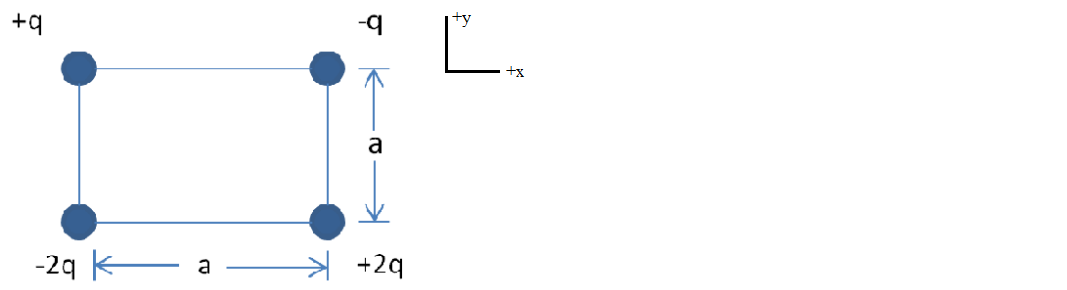# Problem: The four charges form a square of edge length a. What is the direction of the net electric field at the square's center? a. -x b. +x c. +y d. -y e. The field is zero at the center.

🤓 Based on our data, we think this question is relevant for Professor Duke's class at FSU.

###### Problem Details

The four charges form a square of edge length a. What is the direction of the net electric field at the square's center?

a. -x

b. +x

c. +y

d. -y

e. The field is zero at the center.What scientific concept do you need to know in order to solve this problem?

Our tutors have indicated that to solve this problem you will need to apply the Electric Field concept. You can view video lessons to learn Electric Field. Or if you need more Electric Field practice, you can also practice Electric Field practice problems.

What is the difficulty of this problem?

Our tutors rated the difficulty ofThe four charges form a square of edge length a. What is the...as medium difficulty.

How long does this problem take to solve?

Our expert Physics tutor, Juan took 3 minutes and 43 seconds to solve this problem. You can follow their steps in the video explanation above.

What professor is this problem relevant for?

Based on our data, we think this problem is relevant for Professor Duke's class at FSU.